# Osculating circle

(diff) ← Older revision | Latest revision (diff) | Newer revision → (diff)

at a given pointof a curveThe circle that has contact of orderwithat(see Osculation). If the curvature ofatis zero, then the osculating circle degenerates into a straight line. The radius of the osculating circle is called the radius of curvature ofat, and its centre the centre of curvature (see Fig.). Ifis the plane curve given by an equation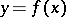, then the radius of the osculating circle is given by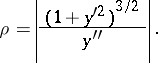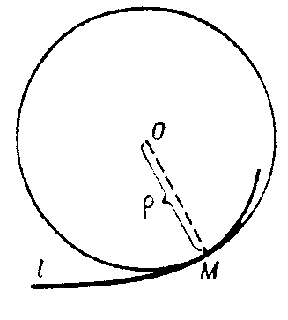Figure: o070540a

Ifis the spatial curve given by equations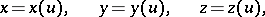then the radius of the osculating circle is given by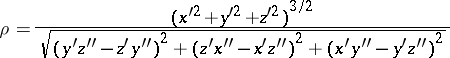(where the primes denote differentiation with respect to).